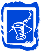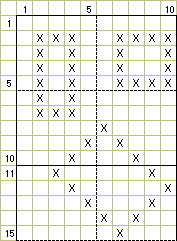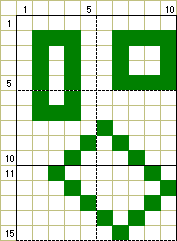## Pattern Guide: Squares & RectanglesRectangles and their even-sided children, Squares, are as easy to plot as straight lines. These straight-sided, right-angled shapes are plotted as pairs of parallel straight lines, whether those sides are horizontal, vertical, or diagonal. Just as horizontal and vertical lines are made easy by the rectangular canvas grid, rectangles and squares that are aligned with the grid are equally easy. Rectangles that are tilted with respect to the canvas need perpendicular (at right angles to each other) diagonal sides. A perpendicular is made by inverting the line-segment pattern used to create the original line. So an [up-1, right-1] line would have a perpendicular line that was [down-1, left-1] (see diagram).Examples